🦉🦉   WOOT WOOT!   RAPIDMINER WISDOM 2020 EARLY BIRD REGISTRATION ENDS FRIDAY DEC 13!   REGISTER NOW!   🦉🦉

# How Rapidminer handle same distance for KNN Algorithm

Member Posts: 7Contributor II
edited August 13 in Help
Maybe I'm rather stupid but I just can't find a satisfying answer: Using the KNN-algorithm,
say k=5. Now I try to classify an unknown object by getting its 5 nearest neighbours.
What to do, if distance is a lot of the same distance..
if after determining the 4 nearest neighbors, the next 2 (or more) nearest objects have the same distance and diferent label? Which object of these 2 or more rapidminer chosen as the 5th nearest neighbor?

I confused.. I try in excel, and the result is diferent with rapidminer for some data.

in case like that, how rapidminer sorting distance ?...
something wrong with my data ?, or rapidminer sorting random if same distance ?

Thanks in advanceTagged:

• Employee, Community Manager, Member Posts: 20Community Manager

https://rapidminer.com/blog/k-nearest-neighbors-laziest-machine-learning-technique/

• Member Posts: 7Contributor II
edited August 14
the link you provided is very useful..

unfortunately this is a new account, and can't attach image or link,

based on what I read in other forums and the links that you provide,
so for KNN there are several ways to handle the same distance..?
looking average distance, or something like that.

and which one is used by rapidminer ? ..

I can't understand and find what kind of algorithm used by rapidminer in determining if the distances are the same?..

hmm..
maybe can be described like this
the results of calculating data testing against data training is :

data training 1st to 4th distance is 0 (count distance 0 is 4)
data training 5th to 10th distance is 1 (count of distance 1 is 6)
data training 11th to 15th distance is 2 (count of distance 2 is 5)
data training 16th to 20th distance is 3 (count of distance 3 is 5)
data training 21st to 25th distance is 4 (count of distance 4 is 5)

if the distance is sorted ascending, the result is so many same distance like that.
if k = 5
so in classification, the majority of labels from the data training will be used, which has the lowest 5 distance calculation..

in the rapidminer algorithm what do the majority of the 1st to 5th data labels use? I think not, because there are some different data when I compare it with manual calculations using MS Excel.

or is the majority of the 1st to 25th data labels?
beacause
the distance 0 is 1st
distance 1 is 2nd
distance 2 is 3rd
distance 3 is 4th
distance 4 is 5th

or is there averaged?
or is there another algorithm used by rapidminer?
and the result is different again if weighted vote is checked.

I have not found a suitable rapidminer calculation with my manual calculations with the distance as above.

I hope you understand what I mean..
• Employee, Community Manager, Member Posts: 20Community Manager
Hi @ademuchlis I have promoted you, so you can now post images and screenshots!
• Member Posts: 7Contributor II
edited August 19

as I explained before
actually the problem is like this :

there are 7000 test data training
and 3000 data testing.
and so many same distance..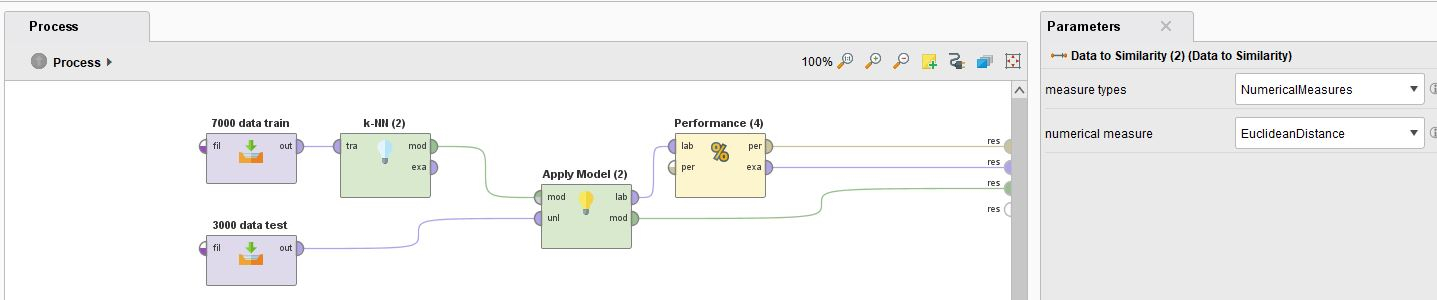I confused.. I try in excel for sorting distance the result is different with rapidminer for some data. in excel the result label is "LU" for K = 5
I tried to train data with ID 182. column A and B is ID.
the calculation is only from column C until L
with label is column M.

results from excel like this, the majority of the labels is "LU" :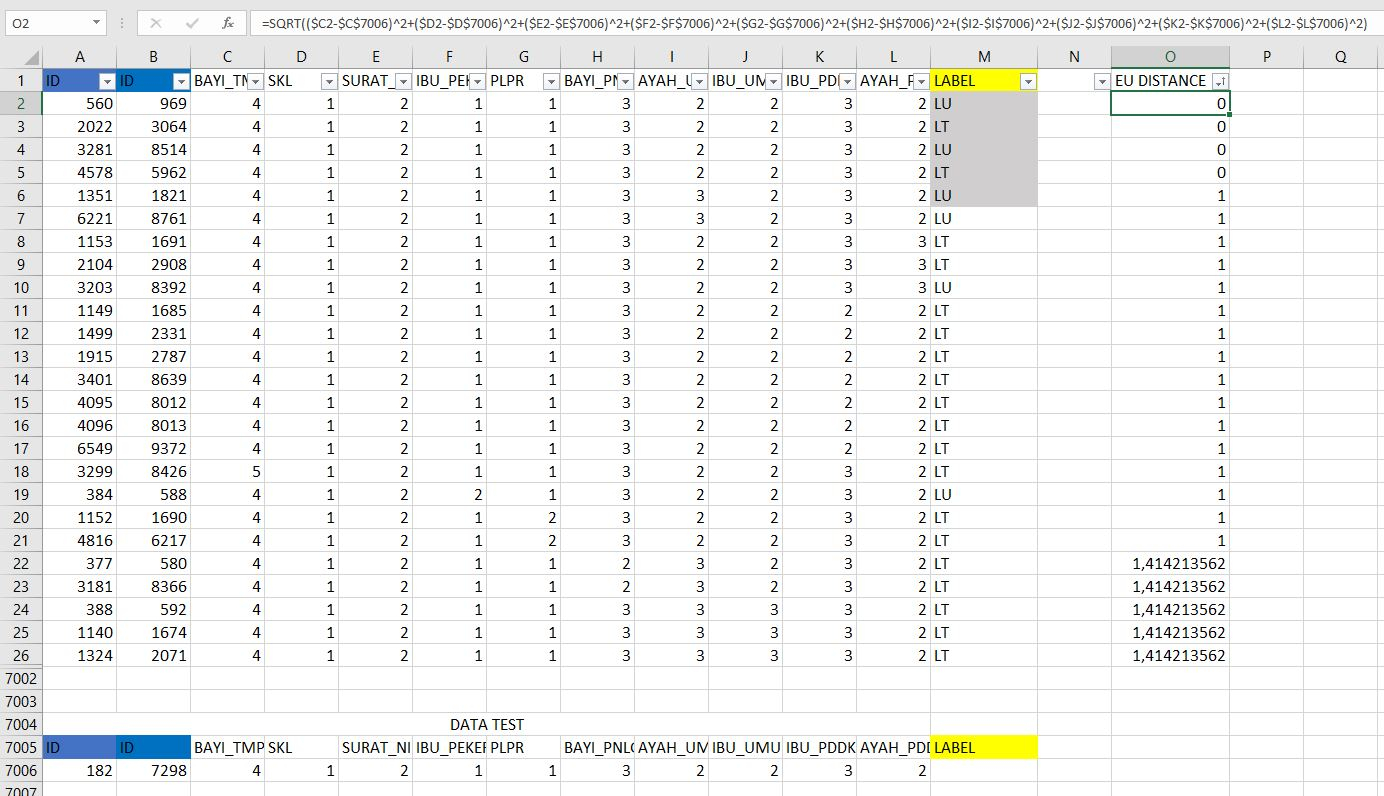result rapidminer weighted vote is checked is "LU" :How rapidminer handle with case like that...

how rapidminer sorting the same distance ?...
something wrong with my data ?,
or rapidminer sorting random for same distance?

• Member Posts: 7Contributor II
edited August 19
• Moderator, RapidMiner Certified Analyst, Member Posts: 909Unicorn

In order we can reproduce what you observe, and understand what's going on, can you please share :

Unfortunately, I have no exact answer to your question....But in first ,approximation, considering k = 5, with no weighted vote :
You have for the four first closer neighbours 2 "LT" and 2 "LU" ...
...but for the fifth closer neighbour there is a lot of candidates which have the same distance to your test point (distance  = 1).
My hypothese, in RapidMiner for the final choice of this fifth closer neighbour and thus for the final choice of the label of the test point are :
- the fifth neightbor is chosen randomly among the candidates (which have all a distance of 1 to the test point).
- if the probability of the 2 labels are the same (here 50% (LT) / 50%(LU)), then the first training point in the dataset, in the loop of the internal code of RapidMiner, is chosen. In other words, it is equivalent to a random choice.
- For equivalent candidates, the candidate are in alphabetic order classified so the "LT" label is chosen instead of "LU" label.
- and finally the more logical explanation from my point of view : there is a majority of label "LT" (and a minority of label "LU") in the candidates of the fifth closer neighbour (which have all a distance of 1 to the test point). So logically the final conclusion is label = "LT" for the test point...

Maybe some RapidMiner's developer(s) can dispel this mystery....?
Thanks you,

Regards,

Lionel
• Administrator, Moderator, Employee, RapidMiner Certified Analyst, RapidMiner Certified Expert, Community Manager, RMResearcher, Member, University Professor Posts: 1,706RM Founder
TBH, I just had a VERY brief look into the relevant classes myself and it was not immediately obvious.  My hunch is that for the fifth neighbor the selection simply is based on the order in which the data points have been added to the queue, i.e. the first data point with the (same) minimal distance will be returned.  In your case that seems to be a LU case.  You could verify by shuffling the order of data points in your data (e.g. by sorting in ascending or descending order before loading the data).  I did not see any reference to random numbers so I would rule out those options...
Hope this helps,
Ingo
RapidMiner Wisdom 2020
February 11th and 12th 2020 in Boston, MA, USA

• Member Posts: 7Contributor II

the following are the results of xml export

<?xml version="1.0" encoding="UTF-8"?><process version="9.2.000">
<context>
<input/>
<output/>
<macros/>
</context>
<operator activated="true" class="process" compatibility="9.2.000" expanded="true" name="Process">
<parameter key="logverbosity" value="init"/>
<parameter key="random_seed" value="2001"/>
<parameter key="send_mail" value="never"/>
<parameter key="process_duration_for_mail" value="30"/>
<parameter key="encoding" value="SYSTEM"/>
<process expanded="true">
<operator activated="true" class="read_csv" compatibility="9.2.000" expanded="true" height="68" name="7000 data training" width="90" x="112" y="34">
<parameter key="csv_file" value="E:\SKRIPSI\2019\HASIL\ayo\data\hasilapp\data_training.csv"/>
<parameter key="column_separators" value=";"/>
<parameter key="trim_lines" value="true"/>
<parameter key="use_quotes" value="true"/>
<parameter key="quotes_character" value="&quot;"/>
<parameter key="escape_character" value="\"/>
<parameter key="comment_characters" value="#"/>
<parameter key="starting_row" value="1"/>
<parameter key="parse_numbers" value="true"/>
<parameter key="decimal_character" value="."/>
<parameter key="grouped_digits" value="false"/>
<parameter key="grouping_character" value=","/>
<parameter key="infinity_representation" value=""/>
<parameter key="date_format" value=""/>
<parameter key="first_row_as_names" value="true"/>
<list key="annotations"/>
<parameter key="time_zone" value="SYSTEM"/>
<parameter key="locale" value="English (United States)"/>
<parameter key="encoding" value="windows-1252"/>
<list key="data_set_meta_data_information">
<parameter key="0" value="ï»¿RN.false.integer.id"/>
<parameter key="1" value="NO_DATA.true.integer.id"/>
<parameter key="2" value="BAYI_TMPT_KLHR.true.integer.attribute"/>
<parameter key="3" value="SKL.true.integer.attribute"/>
<parameter key="4" value="SURAT_NIKAH.true.integer.attribute"/>
<parameter key="5" value="IBU_PEKERJAAN.true.integer.attribute"/>
<parameter key="6" value="PLPR.true.integer.attribute"/>
<parameter key="7" value="BAYI_PNLG_KLHR.true.integer.attribute"/>
<parameter key="8" value="AYAH_UMUR.true.integer.attribute"/>
<parameter key="9" value="IBU_UMUR.true.integer.attribute"/>
<parameter key="10" value="IBU_PDDK_AKHIR.true.integer.attribute"/>
<parameter key="11" value="AYAH_PDDK_AKHIR.true.integer.attribute"/>
<parameter key="12" value="KET_LAPOR.true.polynominal.label"/>
</list>
<parameter key="datamanagement" value="double_array"/>
<parameter key="data_management" value="auto"/>
</operator>
<operator activated="true" class="k_nn" compatibility="9.2.000" expanded="true" height="82" name="k-NN (2)" width="90" x="447" y="34">
<parameter key="k" value="5"/>
<parameter key="weighted_vote" value="true"/>
<parameter key="measure_types" value="NumericalMeasures"/>
<parameter key="mixed_measure" value="MixedEuclideanDistance"/>
<parameter key="nominal_measure" value="NominalDistance"/>
<parameter key="numerical_measure" value="EuclideanDistance"/>
<parameter key="divergence" value="GeneralizedIDivergence"/>
<parameter key="kernel_gamma" value="1.0"/>
<parameter key="kernel_sigma1" value="1.0"/>
<parameter key="kernel_sigma2" value="0.0"/>
<parameter key="kernel_sigma3" value="2.0"/>
<parameter key="kernel_degree" value="3.0"/>
<parameter key="kernel_shift" value="1.0"/>
<parameter key="kernel_a" value="1.0"/>
<parameter key="kernel_b" value="0.0"/>
</operator>
<operator activated="true" class="read_csv" compatibility="9.2.000" expanded="true" height="68" name="3000 data testing" width="90" x="313" y="136">
<parameter key="csv_file" value="E:\SKRIPSI\2019\HASIL\ayo\data\hasilapp\data_testing.csv"/>
<parameter key="column_separators" value=";"/>
<parameter key="trim_lines" value="false"/>
<parameter key="use_quotes" value="true"/>
<parameter key="quotes_character" value="&quot;"/>
<parameter key="escape_character" value="\"/>
<parameter key="comment_characters" value="#"/>
<parameter key="starting_row" value="1"/>
<parameter key="parse_numbers" value="true"/>
<parameter key="decimal_character" value="."/>
<parameter key="grouped_digits" value="false"/>
<parameter key="grouping_character" value=","/>
<parameter key="infinity_representation" value=""/>
<parameter key="date_format" value=""/>
<parameter key="first_row_as_names" value="true"/>
<list key="annotations"/>
<parameter key="time_zone" value="SYSTEM"/>
<parameter key="locale" value="English (United States)"/>
<parameter key="encoding" value="windows-1252"/>
<list key="data_set_meta_data_information">
<parameter key="0" value="ï»¿RN.false.integer.attribute"/>
<parameter key="1" value="NO_DATA.true.integer.id"/>
<parameter key="2" value="BAYI_TMPT_KLHR.true.integer.attribute"/>
<parameter key="3" value="SKL.true.integer.attribute"/>
<parameter key="4" value="SURAT_NIKAH.true.integer.attribute"/>
<parameter key="5" value="IBU_PEKERJAAN.true.integer.attribute"/>
<parameter key="6" value="PLPR.true.integer.attribute"/>
<parameter key="7" value="BAYI_PNLG_KLHR.true.integer.attribute"/>
<parameter key="8" value="AYAH_UMUR.true.integer.attribute"/>
<parameter key="9" value="IBU_UMUR.true.integer.attribute"/>
<parameter key="10" value="IBU_PDDK_AKHIR.true.integer.attribute"/>
<parameter key="11" value="AYAH_PDDK_AKHIR.true.integer.attribute"/>
<parameter key="12" value="KET_LAPOR.true.polynominal.label"/>
</list>
<parameter key="datamanagement" value="double_array"/>
<parameter key="data_management" value="auto"/>
</operator>
<operator activated="true" class="apply_model" compatibility="9.2.000" expanded="true" height="82" name="Apply Model (2)" width="90" x="648" y="136">
<list key="application_parameters"/>
<parameter key="create_view" value="false"/>
</operator>
<operator activated="true" class="performance" compatibility="9.2.000" expanded="true" height="82" name="Performance (4)" width="90" x="782" y="34">
<parameter key="use_example_weights" value="true"/>
</operator>
<connect from_op="7000 data training" from_port="output" to_op="k-NN (2)" to_port="training set"/>
<connect from_op="k-NN (2)" from_port="model" to_op="Apply Model (2)" to_port="model"/>
<connect from_op="3000 data testing" from_port="output" to_op="Apply Model (2)" to_port="unlabelled data"/>
<connect from_op="Apply Model (2)" from_port="labelled data" to_op="Performance (4)" to_port="labelled data"/>
<connect from_op="Apply Model (2)" from_port="model" to_port="result 3"/>
<connect from_op="Performance (4)" from_port="performance" to_port="result 1"/>
<connect from_op="Performance (4)" from_port="example set" to_port="result 2"/>
<portSpacing port="source_input 1" spacing="0"/>
<portSpacing port="sink_result 1" spacing="0"/>
<portSpacing port="sink_result 2" spacing="0"/>
<portSpacing port="sink_result 3" spacing="0"/>
<portSpacing port="sink_result 4" spacing="0"/>
</process>
</operator>
</process>

I only export the process to xml, is that right?

xml, data training, data testing and excel manual calculation attached..
column 1 and 2 is ID, and last column is label.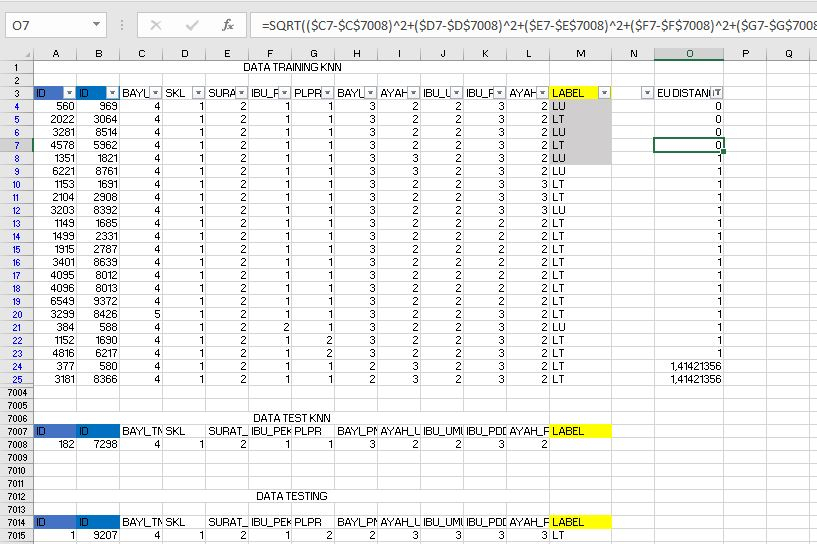I hope the manual excel calculation is easy to understand

• Member Posts: 7Contributor II
Hi @IngoRM

I have shuffled the order of the data, and the results are very different from the one above. the accuracy is different.
even though I'm just shuffling the data order only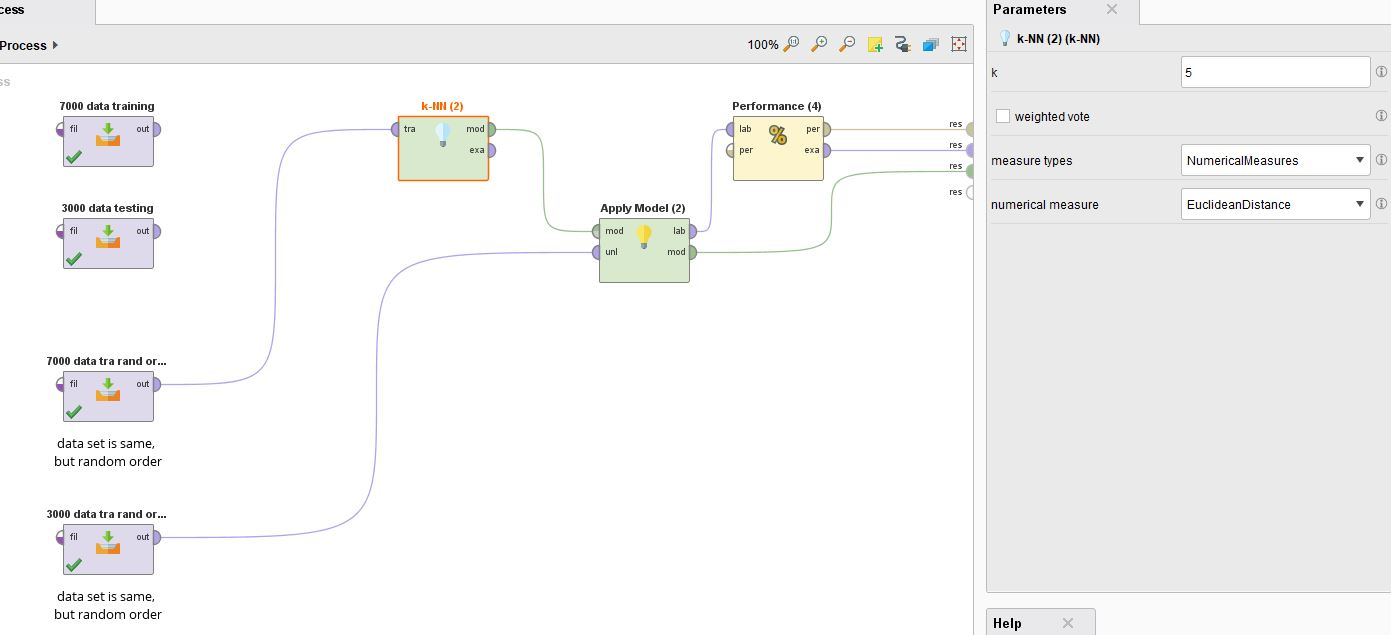if the data has been shuffled. Excel results are also different, because Excel only sort by distance.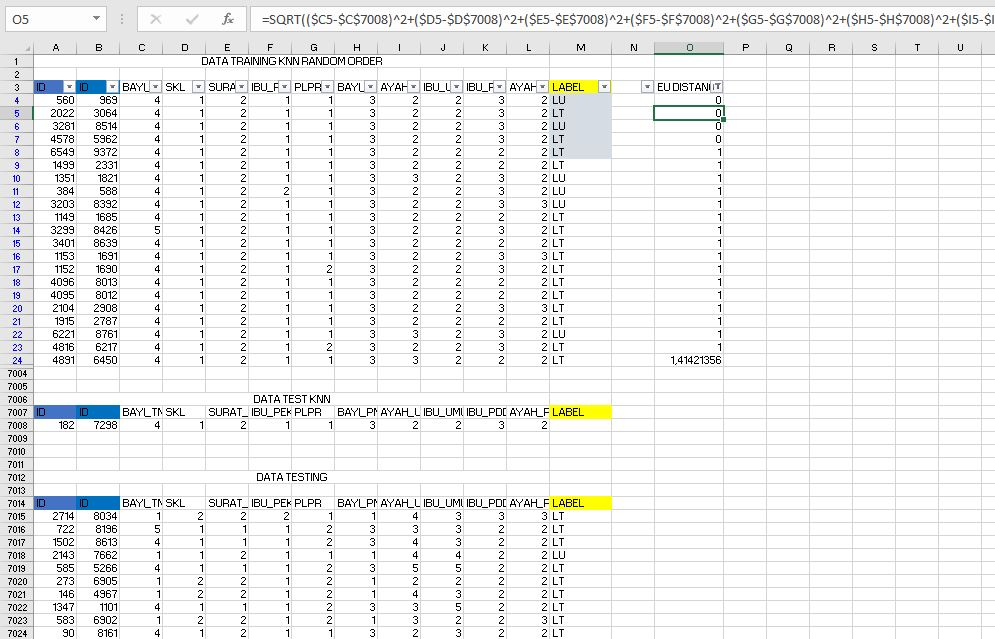is it possible that rapidminer is sorting not only from the distance?

hmm .. so how to calculate this case manually in excel?

initially the data as attached above.

for excel, xml with random order attached here